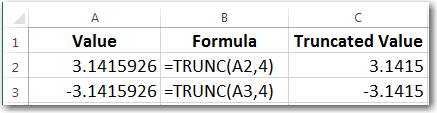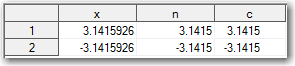Imagine making \$50K a day out of thin air. Did you know that NASDAQ routinely processes around 10,000,000 trades a day? What if instead of rounding cents for each transaction, market makers truncated fractions of cents in the amount they owe you? Under the assumption that each transaction, on average, has half a cent that is usually rounded away, this would produce 10,000,000 x \$0.005 = \$50,000 and nobody would even notice it. I am not saying it's legal, but this example is just an illustration of the power of ordinary truncation.

However, sometimes it is necessary to truncate displayed numeric values to a specified number of decimal places without rounding. For example, if we need to truncate 3.1415926 to 4 decimal places without rounding, the displayed number would be 3.1415 (as compared to the rounded number, 3.1416).

If you think you can truncate numeric values by applying SAS w.d format, think again.

Try running this SAS code:

```data _null_; x=3.1415926; put x= 6.4; run;```

If you expect to get x=3.1415, you will be disappointed. Surprisingly, you will get x=3.1416, which means that SAS format does not truncate the number, but rounds it. Same is true for the DOLLARw.d and COMMAw.d formats.

After running into this problem, I thought to instead use a SAS function to truncate numbers. The TRUNC function comes to mind. Indeed, if you look up the SAS TRUNC function, you will find that it does truncate numeric values, but (surprise!) not to a specified number of decimal places; rather it truncates to a specified number of bytes, which is not the same thing for numerics. This may be useful for evaluating the precision of numeric values, but has no direct bearing on our problem of truncating numeric values to a set number of decimal places.

What turned to be interesting is that the Excel TRUNC function does exactly what we need – it truncates values to a set number of decimal places (removes decimals) without rounding:Truncating numbers in Excel with the TRUNC Function

In general, the technique of number truncation should be limited to reporting purposes when displayed numbers are required to appear truncated. Be careful not to apply truncation before using the numbers in calculations, as you might get some seriously inaccurate results, even worse than when you round numbers before calculations. Unless, of course, your goal is to get inaccurate results, which is quite an honorable goal in fraud detection, simulation and investigation.

I can see two possible solutions to number truncations:

### Solution 1: Numeric truncation

Let’s say we need to truncate the following number X.XXXXXXX , keeping only the red digits (that is get rid of all decimal digits after the 4th decimal place).

We can do it in 3 steps:

1. Multiply our number by 104, effectively making the decimals part of a whole number (shifting the decimal point 4 positions to the right).
2. Apply INT() function that truncates the decimal portion, keeping only the whole portion from the previous step.
3. Divide the result of step 2 by 104, effectively restoring the order disturbed by step 1 (shifting the decimal point 4 positions to the left).

Here is SAS code implementing this algorithm:

```%let d = 4; /* d must be a whole number: 0, 1, 2... */   data _null_; x = 3.1415926; p = 10**&d; y = int(x*p)/p; put x= / y=; run;```

If we run this code SAS log will show the following (expected and desired) results:

x=3.1415926
y=3.1415

WARNING: While in the SAS code example above the int() function might be substituted with the floor() function, for negative numbers the floor() function would produce incorrect results. For negative numbers, the ceil() function is the correct choice. However, the int() function does exactly what we need for both positive and negative numbers.

### Solution 2: Character truncation

Since we use truncated numbers for output only, we can solve our truncation problem by converting numeric value into character, and then use character functions to get rid of extra digits. Let’s solve the same problem, to truncate the following number X.XXXXXXX keeping only the red digits.

Using this character approach we can also do it in 3 steps:

1. Convert the numeric value into a character value and assign it to a new character variable.
2. Determine position of a decimal point in the character string.
3. Sub-string our initial character string to keep only 4 characters after decimal point.

Here is SAS code implementing this algorithm:

```%let d = 4; /* d must be a whole number: 0, 1, 2... */   data _null_; x = 3.1415926; y = put(x,best.); y = substr(y,1,index(y,'.')+&d); put x= / y=; run;```

If we run this code SAS log will show the following results:

x=3.1415926
y=3.1415

As you can see, these results are correct and identical to the results of numeric truncation.

Both numeric and character truncation methods work for positive and negative numbers.

### User-defined functions

We can also implement the above two methods as user-defined functions, say truncn() and truncc(), using PROC FCMP:

```proc fcmp outlib = sasuser.functions.truncations; function truncn (x,d); p = 10**d; y = int(x*p)/p; return(y); endsub;   function truncc (x,d); y = put(x,best.); y = substr(y,1,index(y,'.')+d); return(y); endsub; run;```

Then we can use those user-defined functions truncn() and truncc() as illustrated in the following SAS code sample:

```options cmplib=sasuser.functions;   data A; length x n 8 c \$9; input x; n = truncn(x,4); c = truncc(x,4); datalines; 3.1415926 -3.1415926 run;```

This code will produce the following A dataset:Notice that variables x and n are of numeric type while variable c is of character type.

### Questions

1. Which of these two methods of decimal number truncation do you like more? Why?
2. Does it make sense to use these methods as user-defined functions? Why?

tags: SAS Programmers

Truncating decimal numbers in SAS without rounding was published on SAS Users.